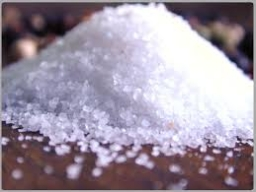# Weight fraction

To prepare the brine, we need 1500 g of an aqueous salt solution with a weight fraction of 0.08. What weight of salt and volume of water do we need to prepare it?

m1 =  120 g
V2 =  1.38 l

### Step-by-step explanation:Did you find an error or inaccuracy? Feel free to write us. Thank you!

Tips to related online calculators
Need help to calculate sum, simplify or multiply fractions? Try our fraction calculator.
Tip: Our Density units converter will help you with the conversion of density units.
Do you want to convert mass units?